##### Helping Kids with Coding For DummiesWhen coding, it’s important to teach kids the basics of setting and finding position. Setting the position of an object means assigning it coordinates to put it in a specific place onscreen. Finding the position of an object means identifying its coordinates to know where it’s located.

## Using pseudocode

While each programming language uses its own structure for setting and finding coordinates, a typical pseudocode expression you may write to set the position of an object looks like this:
```setx <em>x-coordinate</em>
sety <em>y-coordinate</em>```
Or
`setposition <em>(x-coordinate, y-coordinate)</em>`
To find the current position of an object, you can write pseudocode for each separate coordinate: `x-position` for the x-coordinate of the object, and `y-position` for the y-coordinate of the object. You can also write `position` to describe the object position as a coordinate pair.

## Using Scratch to set position

To set the x-coordinate of an object in Scratch, use the `set x to <em>number</em>` command in the Motion category. The minimum value of the x-coordinate ranges is -240, and the maximum value is 240.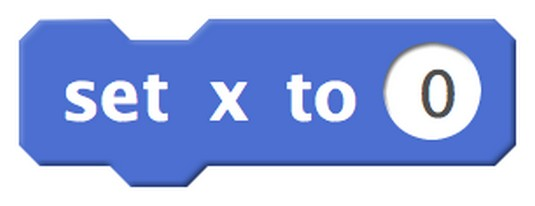Use `set x to number` command to set the x-coordinate of an object in Scratch.

To set the y-coordinate of an object in Scratch, use the `set y to <em>number</em>` command in the Motion category. The minimum value of the y-coordinate ranges is -180, and the maximum value is 180.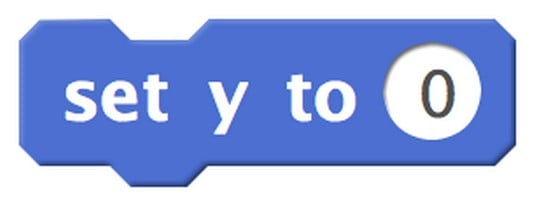Use `set y to number` command to set the y-coordinate of an object in Scratch.

To set both the x-coordinate and y-coordinate of an object in Scratch, use the `go to x: <em>number</em> y: <em>number</em>` command in the Motion category. The range of the x-coordinate value is -240 to 240, and the range of the y-coordinate value is -180 to 180.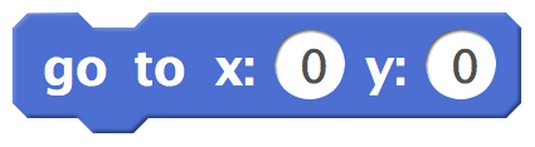Use `go to x: number y: number` command to set the position of an object in Scratch.

In Scratch, you can set the size of an object using the `set size to number %` command in the Looks category. This sets the size of the object as a percentage of its original size. Percentages smaller than 100 shrink the object. Percentages larger than 100 grow the object.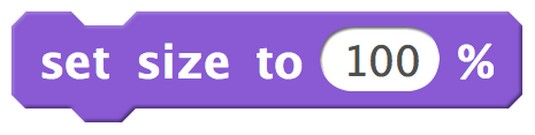Use the `set size to number %` command to set size of an object in Scratch.

## Using Scratch to find position

To find the x-coordinate of an object in Scratch, use the `x position` command in the Motion category. To find the y-coordinate of an object in Scratch, use the `y position` command in the Motion category. You and your coder can use these commands in your programs when you need to write commands that require information about an object's position.

As you code, sometimes you want to position an object (sprite) onscreen and then get its coordinates. You can do this for any sprite using either of these methods:

• Select the checkbox next to the `x position` command and the `y position command` in the Motion category to show these values onscreen.
• On the thumbnail of the sprite, click the “i” icon to expand its information center and then view the x: and y: values displayed there.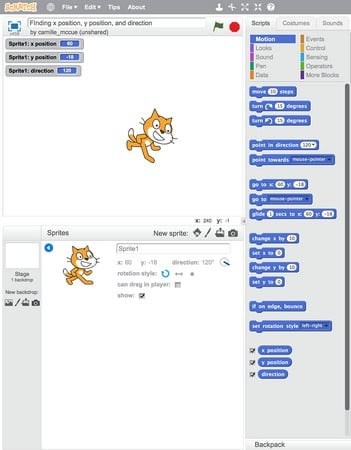Two ways to view the current x- and y-coordinates of a sprite.

In both methods, the coordinates of Scratch Cat are (60, -18).

## Using JavaScript

To set both the x-coordinate and y-coordinate of an object in JavaScript, identify the object you want to position, and then use the `setPosition` command.

Here are the steps for how to position an image of a mouse onscreen in the Code.org App Lab, using JavaScript.

1. In App Lab, click the Design button above the emulator of the mobile device.
2. In the Workspace, select Image →   Choose.

The Choose Assets dialog box opens.

4. Select the file you want, and then click the Choose button. The uploaded file appears in the dialog box. The name of the uploaded image file shown here is `mouse.png`.
5. In the App Lab program workspace, type these commands:
```>image("character", "mouse.png");
setPosition("character", 160, 225, 100, 100);```
Here is what these commands do: The first command creates an image reference identification, `character`, and links the file, `mouse.png`, to this identification. The second command displays the image in `character` according to four quantities: the x-coordinate of the object, the y-coordinate of the object, the width of the object in pixels, and the height of the object in pixels.

In App Lab, the range of the x-coordinate value is 0 to 320, and the range of the y-coordinate value is 0 to 450. When using JavaScript to program images displayed on a webpage, these values have larger maximum values, representing the larger size of a computer screen.

The image below shows the mouse positioned at the coordinates (160, 225), which is the exact center of the screen. You can see that the mouse is positioned by its upper-left corner where the tip of its tail is located. The mouse has a width of 100 pixels and a height of 100 pixels.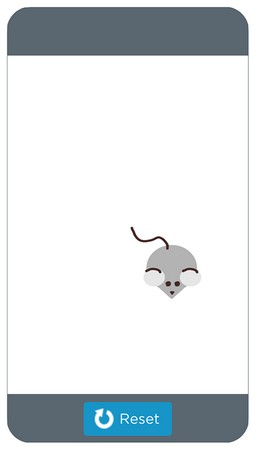Use the `setPosition` command to set the position of an object in JavaScript.

To find the x-coordinate of an object in JavaScript, use the `getXPosition("character");` command where `character` is the identification reference of the object. To find the y-coordinate of an object in JavaScript, use the `getYPosition("character");` command where `character` is the identification reference of the object.

You and your coder can write the following code to find and display onscreen the coordinates of an object named `character`. This assumes you have uploaded an image file and assigned it to the reference identification, `character`. Type this code in the App Lab program workspace.

```var x = getXPosition("character");
var y = getYPosition("character");
textLabel("xcor");
textLabel("ycor");
setText("xcor", "x-coordinate is " + x);
setText("ycor", "y-coordinate is " + y);```
Here is how this code works:
• The `var x` variable gets the x position and the `var y` variable gets the y position of the object.
• The two `textLabel` commands create locations onscreen, called `xcor` and `ycor`, to display information.
• Each `setText` command displays a value in a text label. The value of `x` displays in the `xcor` label and the value of `y` displays in the `ycor`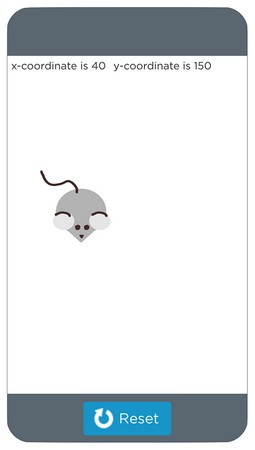Use the `getXPosition` and `getYPosition` commands to get the position of an object in JavaScript.